SBAS438C May   2008  – November 2019

PRODUCTION DATA.

1. Features
2. Applications
3. Description
1.     Device Images
4. Revision History
5. Pin Configuration and Functions
6. Specifications
7. Detailed Description
1. 7.1 Overview
2. 7.2 Functional Block Diagram
3. 7.3 Feature Description
4. 7.4 Device Functional Modes
1. 7.4.1 Serial Interface
1. 7.4.1.1 Input Shift Register
8. Application and Implementation
1. 8.1 Application Information
2. 8.2 Typical Application
1. 8.2.1 DAC9881 Sample-and-Hold Circuit
3. 8.3 System Example
9. Power Supply Recommendations
10. 10Layout
11. 11Device and Documentation Support
12. 12Mechanical, Packaging, and Orderable Information

• RGE|24
• RGE|24

### 6.9 Typical Characteristics: AVDD = 5 V

at TA = 25°C, VREFH = 5 V, VREFL = 0 V, and gain = 1X mode (unless otherwise noted)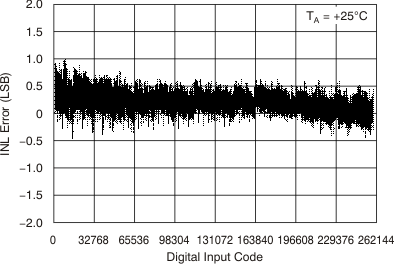Figure 4. Linearity Error vs Digital Input Code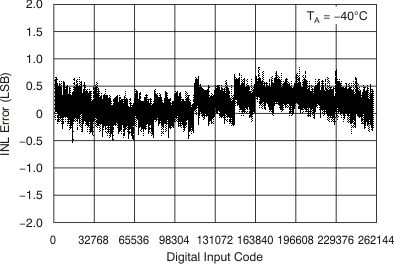Figure 6. Linearity Error vs Digital Input Code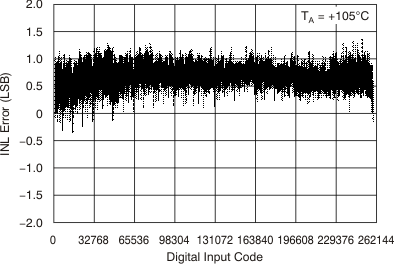Figure 8. Linearity Error vs Digital Input Code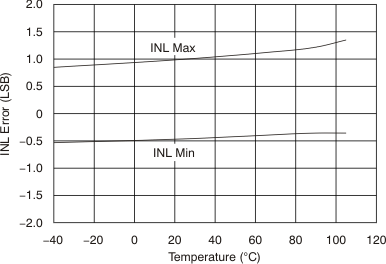Figure 10. Linearity Error vs Temperature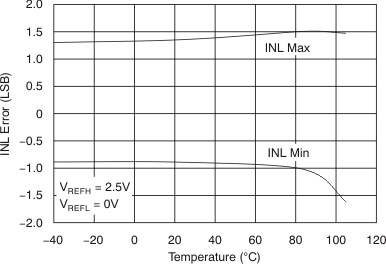Gain = 2X mode
Figure 12. Linearity Error vs Temperature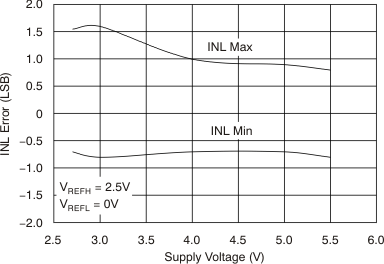Figure 14. Linearity Error vs Supply Voltage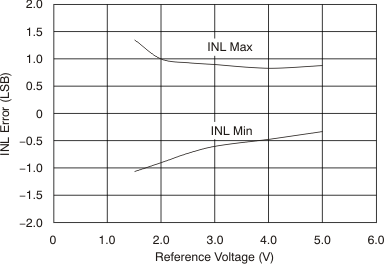Figure 16. Linearity Error vs Reference Voltage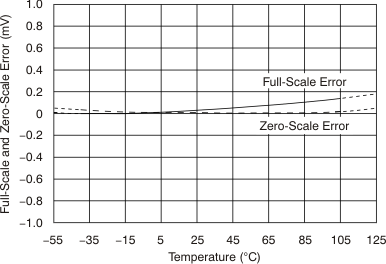Figure 18. Full-Scale and Zero-Scale Error vs Temperature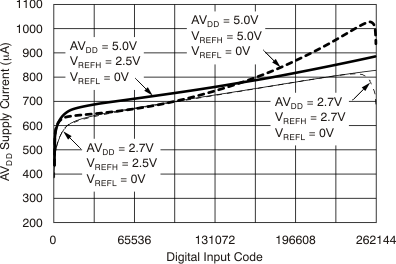Figure 20. AVDD Supply Current vs Digital Input Code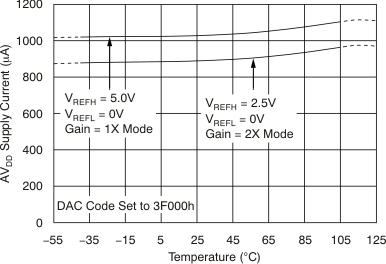Figure 22. AVDD Supply Current vs Temperature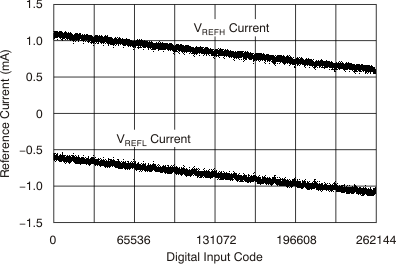Figure 24. Reference Current vs Digital Input Code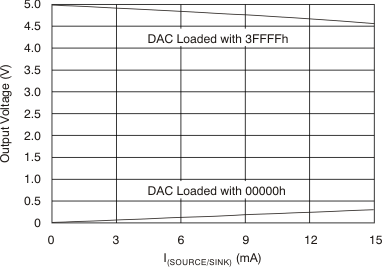Figure 26. Output Voltage vs Drive Current CapabilityFigure 28. Output Voltage vs Drive Current Capability (Operation Near AGND Rail)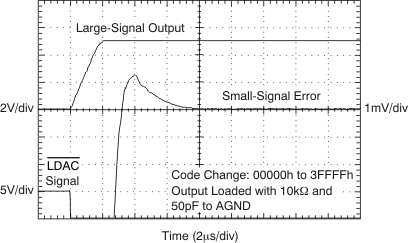Figure 30. Large-Signal Settling Time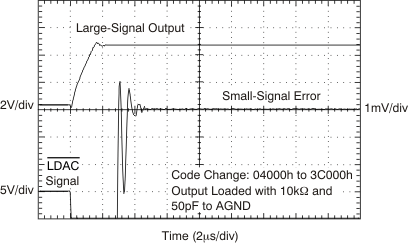Figure 32. Large-Signal Settling Time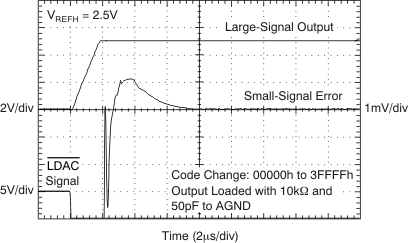Gain = 2X mode
Figure 34. Large-Signal Settling Time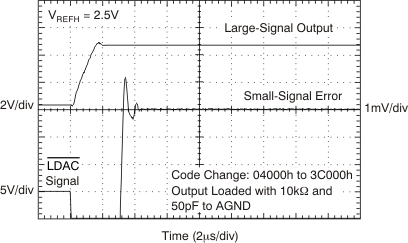Gain = 2X mode
Figure 36. Large-Signal Settling Time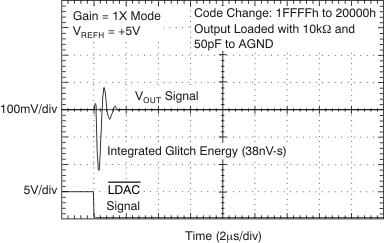Figure 38. Major Carry Glitch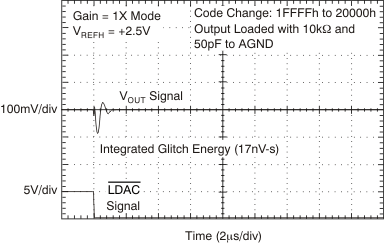Figure 40. Major Carry Glitch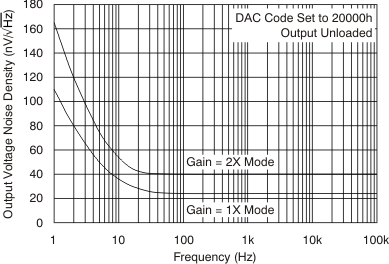Figure 42. Output Noise Density vs Frequency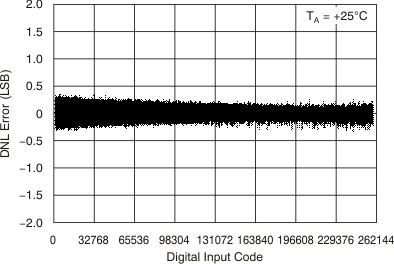Figure 5. Differential Linearity Error vs Digital Input Code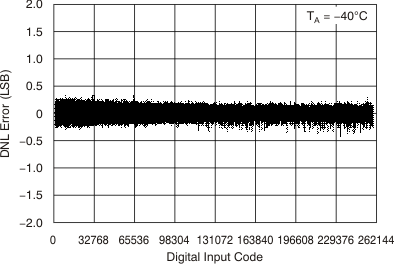Figure 7. Differential Linearity Error vs Digital Input Code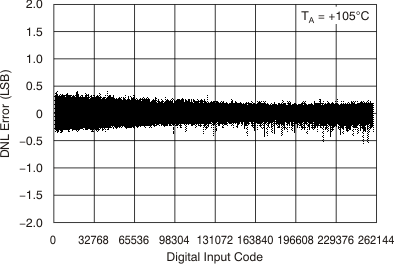Figure 9. Differential Lineary Error vs Digital Input Code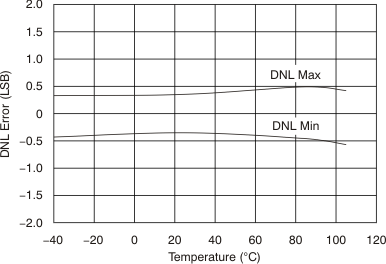Figure 11. Differential Linearity Error vs Temperature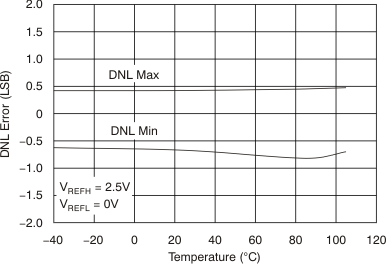Gain = 2X mode
Figure 13. Differential Linearity Error vs Temperature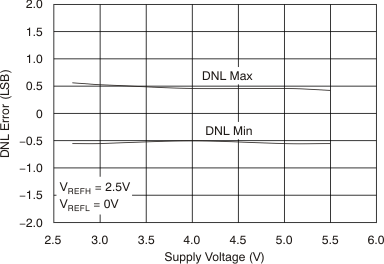Figure 15. Differential Linearity Error vs Supply Voltage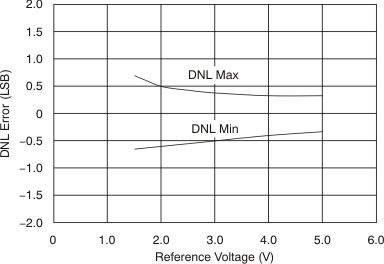Figure 17. Differential Linearity Error vs Reference Voltage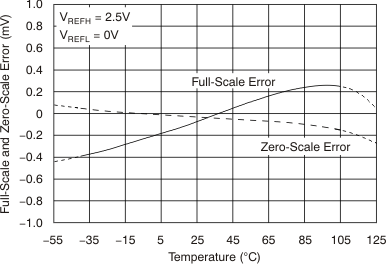Gain = 2X mode
Figure 19. Full-Scale and Zero-Scale Error vs Temperature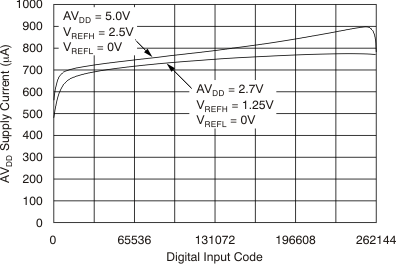Gain = 2X mode
Figure 21. AVDD Supply Current vs Digital Input Code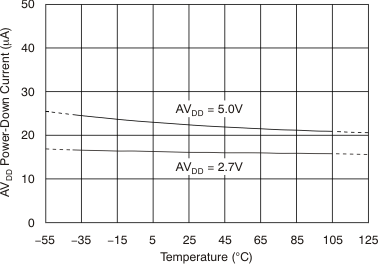Figure 23. AVDD Power-Down Current vs Temperature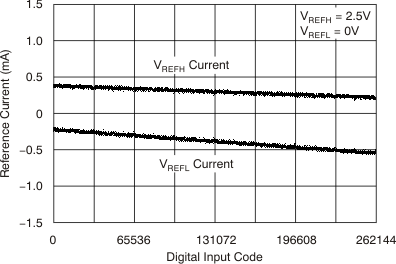Gain = 2X mode
Figure 25. Reference Current vs Digital Input Code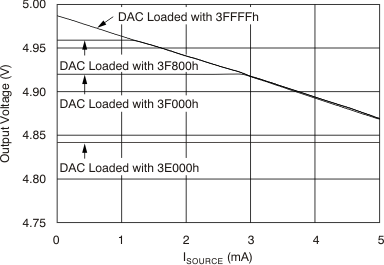Figure 27. Output Voltage vs Drive Current Capability (Operation Near AVDD Rail)Figure 29. IOVDD Supply Current vs Logic Input Voltage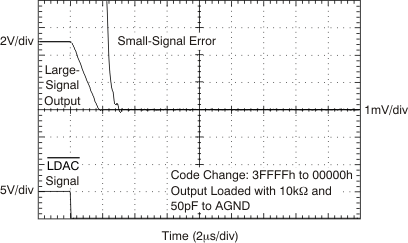Figure 31. Large-Signal Settling TimeFigure 33. Large-Signal Settling Time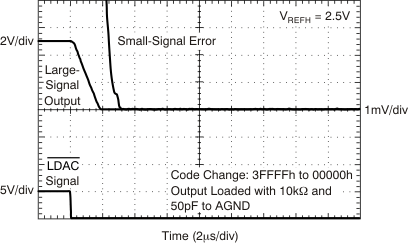Gain = 2X mode
Figure 35. Large-Signal Settling Time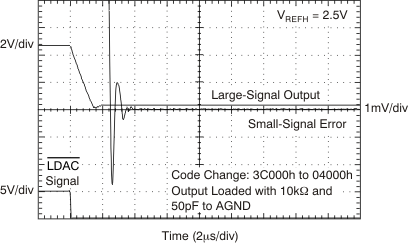Gain = 2X mode
Figure 37. Large-Signal Settling Time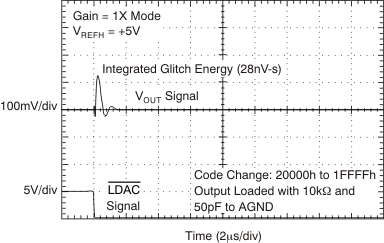Figure 39. Major Carry Glitch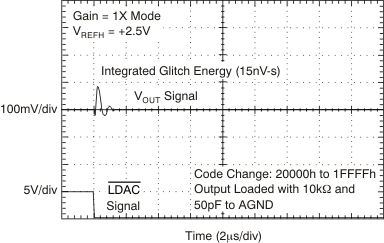Figure 41. Major Carry Glitch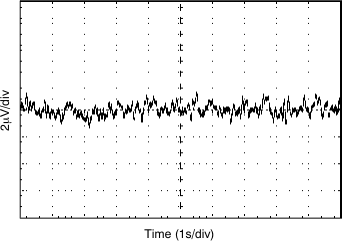Figure 43. Low-Frequency Output Noise (0.1 Hz to 10 Hz)